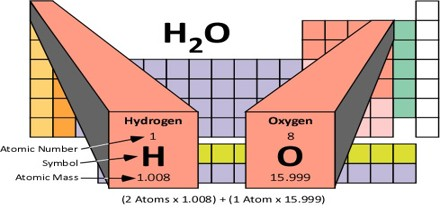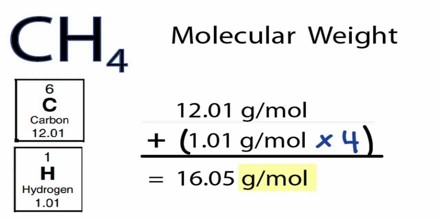Molecular Mass

Definition

Molecular Mass is the amount of mass associated with a molecule. It is also called as molecular weight. It can be calculated by adding the mass of each atom multiplied by the number of atoms of the element present in the molecule. The molecular mass gives the mass of a molecule relative to that of the 12C atom, which is taken to have a mass of 12. Molecular mass is a dimensionless quantity, but it is given the unit Dalton or atomic mass unit as a means of indicating the mass is relative to 1/12th the mass of a single atom of carbon-12.Molecular Mass of a substance is the mass of all the atoms in a given formula, in atomic mass units (amu). So just like we added up the weight of all the family members to get the total weight of the family, we can add up the atomic weights (or masses) of all the atoms in a molecule to determine the molecular mass of the molecule. In the same way, we can calculate the molar mass of a compound, using the molar masses of all the atoms in units of grams per mole. That’s because the molar mass of a substance is the mass of all the atoms in a given formula, in units of grams per mole (g/mol).

The molecular mass or weight of molecules can be determined by following methods:

• Mass Spectrometry: This method is generally used in determining the mass of small molecules. This is reported as monoisotropic mass. A theoretical average molecular mass can be calculated using the relative atomic masses found on a typical periodic table, since there is likely to be a statistical distribution of atoms representing the isotopes throughout the molecule. This however may differ from the true average molecular mass of the sample due to natural (or artificial) variations in the isotopic distributions.
• Hydrodynamic Method: The weight is determined as per Mark-Houwink relations. This method requires calibration hence it is also described as relative molecular weight determination method.
• Static Light Scattering: Molecular weight is determined from the amount of light scattered using the Zimm method. Molecular weights determined by this method do not require calibration, hence the term “absolute”. The only external measurement required is refractive index increment, which describes the change in refractive index with concentration.Calculation of Molecular Mass

Molecular mass may be calculated by taking the atomic mass of each element present and multiplying it by the number of atoms of that element in the molecular formula. Then, the number of atoms of each element is added together.

For example, to find the molecular mass of methane, CH4, the first step is to look up the atomic masses of carbon C and hydrogen H using a periodic table:

Carbon Atomic Mass = 12.011

Hydrogen Atomic Mass = 1.00794

Because there is no subscript following the C, we know there is only one carbon atom present in methane. The subscript 4 following the H means there are four atoms of hydrogen in the compound.

So, adding up the atomic masses, we get:

Methane Molecular Mass = sum of carbon atomic masses + sum of hydrogen atomic masses

Methane Molecular Mass = 12.011 + (1.00794)(4)

Methane Atomic Mass = 16.043

This value may be reported as a decimal number or as 16.043 Da or 16.043 amu.

Note the number of significant digits in the final value.

The correct answer uses the smallest number of significant digits in the atomic masses, which in this case is the number in the atomic mass of carbon.

The molecular mass of C2H6 is approximately 30 or [(2 x 12) + (6 x 1)]. Therefore the molecule is about 2.5 times as heavy as the 12C atom or about the same mass as the NO atom with a molecular mass of 30 or (14+16).